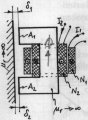#### thexy

Joined Dec 13, 2015
130
In the approximately ideal magnetizable iron circle with two different air gaps two coils are arranged concentrically. Calculate the mutual inductance without regard to the scattering.I have results but I don't understand some parts so if someone would like to help me, I would be thankful.
First they use Ampere's law:

$$B_1\delta _1+B_2\delta _2=\mu_0N_1I_1$$
I don't understand this at all, why this sum, why those formulas?
Than Gauss's law:
$$\Phi =B_1A_1=B_2A_S$$
I understand this part.

And than they use:
$$\Phi_{v2} = -\Phi N_2$$
From where comes this minus?

#### MrAl

Joined Jun 17, 2014
10,599
Hello,

Did you try this problem using reluctance instead? Often comes out much simpler.
I'd have to look that up again myself, but you could try that yourself first.